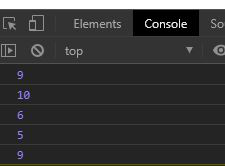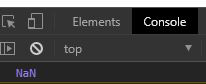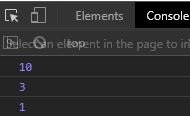Open in App
Not now

# Underscore.js _.random() Function

• Last Updated : 14 Jul, 2020

Underscore.js is a library in javascript that makes operations on arrays, string, objects much easier and handy.
The _.random() function is used to return a random integer which is in the range provided to the function.

Note: It is very necessary to link the underscore CDN before going and using underscore functions in the browser. When linking the underscore.js CDN The “_” is attached to the browser as a global variable.

Syntax:

`_.random(min, max);`

Parameters: It takes the following parameters.

• min value: It is the minimum random value to be returned by the function.
• max value: It is the maximum random value that can be returned by the function.

Return Value: It returns a random integer between the min and max. If only one value is passed then it will return random value between 0 and passed value.

Example 1:

 ` ` `<``html``> ` ` `  `<``head``> ` `    ``<``script` `src``= ` `"https://cdnjs.cloudflare.com/ajax/libs/underscore.js/1.9.1/underscore-min.js"``> ` `    `` ` ` ` ` `  `<``body``> ` `    ``<``script``> ` `        ``let min = 5; ` `        ``let max = 10; ` ` `  `        ``// Printing 5 random values ` `        ``// in range 5 and 10 ` `        ``for (let i = 0; i < ``5``; i++) { ` `            ``console.log(_.random(min, max)); ` `        ``} ` `    `` ` ` ` ` `  ` `

Output:Example 2: If no parameter is given to the random function.

 ` ` `<``html``> ` ` `  `<``head``> ` `    ``<``script` `src``= ` `"https://cdnjs.cloudflare.com/ajax/libs/underscore.js/1.9.1/underscore-min.js"``> ` `    `` ` ` ` ` `  `<``body``> ` `    ``<``script``> ` ` `  `        ``// Without passing any  ` `        ``// value to function ` `        ``console.log(_.random()) ` `    `` ` ` ` ` `  ` `

Output:Example 3: When only one parameter is given then random value is in range 0 to n, where n is the parameter given.

 ` ` `<``html``> ` ` `  `<``head``> ` `    ``<``script` `src``= ` `"https://cdnjs.cloudflare.com/ajax/libs/underscore.js/1.9.1/underscore-min.js"``> ` `    `` ` ` ` ` `  `<``body``> ` `    ``<``script``> ` `        ``console.log(_.random(10)) ` `        ``console.log(_.random(5)) ` `        ``console.log(_.random(8)) ` `    `` ` ` ` ` `  ` `

Output:My Personal Notes arrow_drop_up
Related Articles